# Quantum Numbers

A total of four quantum numbers are used to describe completely the movement and trajectories of each electron within an atom. The combination of all quantum numbers of all electrons in an atom is described by a wave function that complies with the Schrödinger equation. Each electron in an atom has a unique set of quantum numbers; according to the Pauli Exclusion Principle, no two electrons can share the same combination of four quantum numbers. Quantum numbers are important because they can be used to determine the electron configuration of an atom and the probable location of the atom’s electrons. Quantum numbers are also used to determine other characteristics of atoms, such as ionization energy and the atomic radius.

## The Four Electronic Quantum Numbers

Quantum numbers designate specific shells, subshells, orbitals, and spins of electrons. This means that they describe completely the characteristics of an electron in an atom, i.e., they describe each unique solution to the Schrödinger equation, or the wave function, of electrons in an atom. There are a total of four quantum numbers: the principal quantum number (n), the orbital angular momentum quantum number (l), the magnetic quantum number (ml), and the electron spin quantum number (ms). The principal quantum number, $n$, describes the energy of an electron and the most probable distance of the electron from the nucleus. In other words, it refers to the size of the orbital and the energy level an electron is placed in. The number of subshells, or $l$, describes the shape of the orbital. It can also be used to determine the number of angular nodes. The magnetic quantum number, ml, describes the energy levels in a subshell, and ms refers to the spin on the electron, which can either be up or down.

### The Principal Quantum Number (n)

The principal quantum number, n, designates the principal electron shell. Because n describes the most probable distance of the electrons from the nucleus, the larger the number n is, the farther the electron is from the nucleus, the larger the size of the orbital, and the larger the atom is. n can be any positive integer starting at 1, as n=1 designates the first principal shell (the innermost shell). The first principal shell is also called the ground state, or lowest energy state. This explains why n can not be 0 or any negative integer, because there exists no atoms with zero or a negative amount of energy levels/principal shells. When an electron is in an excited state or it gains energy, it may jump to the second principle shell, where . This is called absorption because the electron is “absorbing” photons, or energy. Known as emission, electrons can also “emit” energy as they jump to lower principle shells, where n decreases by whole numbers. As the energy of the electron increases, so does the principal quantum number, e.g., n = 3 indicates the third principal shell, n = 4 indicates the fourth principal shell, and so on.

n=1,2,3,4…

### Example

If n = 7, what is the principal electron shell?

### Example

If an electron jumped from energy level n = 5 to energy level n = 3, did absorption or emission of a photon occur?

### The Orbital Angular Momentum Quantum Number (l)

The orbital angular momentum quantum number l determines the shape of an orbital, and therefore the angular distribution. The number of angular nodes is equal to the value of the angular momentum quantum number $l$. (For more information about angular nodes, see Electronic Orbitals.) Each value of l indicates a specific s, p, d, f subshell (each unique in shape.) The value of l is dependent on the principal quantum number n. Unlike n, the value of l can be zero. It can also be a positive integer, but it cannot be larger than one less than the principal quantum number (n-1):

l=0, 1, 2, 3, 4…, (n-1)

### Example

If n = 7, what are the possible values of l?

### Example

If l = 4, how many angular nodes does the atom have?

### The Magnetic Quantum Number (ml)

The magnetic quantum number ml determines the number of orbitals and their orientation within a subshell. Consequently, its value depends on the orbital angular momentum quantum number l. Given a certain l, ml is an interval ranging from –l to +l, so it can be zero, a negative integer, or a positive integer.

ml=l, (-l +1),( -l +2),…, -2, -1, 0, 1, 2, … (l – 1), (l – 2), +l

### Examples

If n=3, and l=2, then what are the possible values of ml ?

### The Electron Spin Quantum Number (ms)

Unlike n, l, and ml, the electron spin quantum number ms does not depend on another quantum number. It designates the direction of the electron spin and may have a spin of +1/2, represented by↑, or –1/2, represented by ↓. This means that when ms is positive the electron has an upward spin, which can be referred to as “spin up.” When it is negative, the electron has a downward spin, so it is “spin down.” The significance of the electron spin quantum number is its determination of an atom’s ability to generate a magnetic field or not. (Electron Spin.)

ms± ½

### Example

List the possible combinations of all four quantum numbers when n=2, l=1, and ml=0

### Example

Can an electron with ms=1/2 have a downward spin?

## A Closer Look at Shells, Subshells, and Orbitals

### Principal Shells

The value of the principal quantum number n is the level of the principal electronic shell (principal level). All orbitals that have the same n value are in the same principal level. For example, all orbitals on the second principal level have a principal quantum number of n=2. When the value of n is higher, the number of principal electronic shells is greater. This causes a greater distance between the farthest electron and the nucleus. As a result, the size of the atom and its atomic radius increases.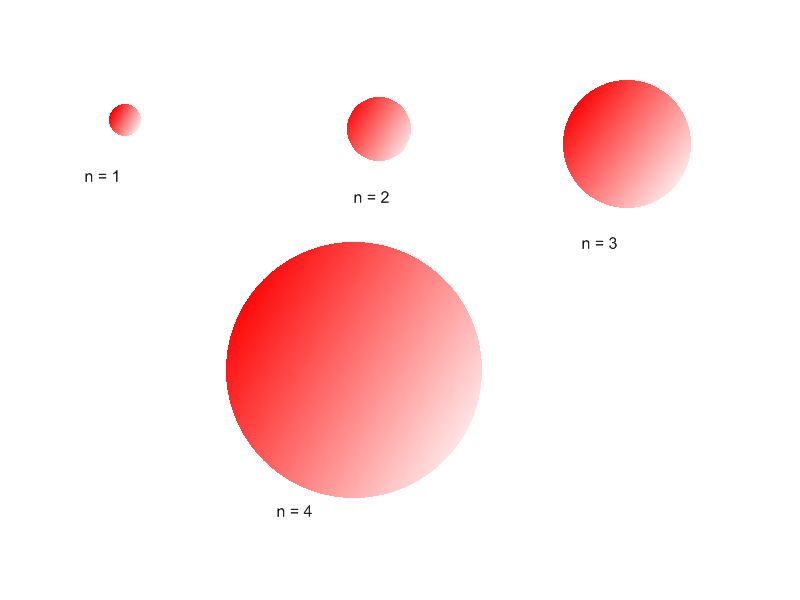Because the atomic radius increases, the electrons are farther from the nucleus. Thus it is easier for the atom to expel an electron because the nucleus does not have as strong a pull on it, and the ionization energy decreases.

### Example

Which orbital has a higher ionization energy, one with (n=3) or (n=2)?

### Subshells

The number of values of the orbital angular number l can also be used to identify the number of subshells in a principal electron shell:

• When n = 1, l= 0 (l takes on one value and thus there can only be one subshell)
• When n = 2, l= 0, 1 (l takes on two values and thus there are two possible subshells)
• When n = 3, l= 0, 1, 2 (l takes on three values and thus there are three possible subshells)

After looking at the examples above, we see that the value of n is equal to the number of subshells in a principal electronic shell:

• Principal shell with n = 1 has one subshell
• Principal shell with n = 2 has two subshells
• Principal shell with n = 3 has three subshells

To identify what type of possible subshells n has, these subshells have been assigned letter names. The value of l determines the name of the subshell:

Name of Subshell Value of l
s subshell 0
p subshell 1
d subshell 2
f subshell 3

Therefore:

• Principal shell with n = 1 has one s subshell (l = 0)
• Principal shell with n = 2 has one s subshell and one p subshell (l = 0, 1)
• Principal shell with n = 3 has one s subshell, one p subshell, and one d subshell (l = 0, 1, 2)

We can designate a principal quantum number, n, and a certain subshell by combining the value of n and the name of the subshell (which can be found using l). For example, 3p refers to the third principal quantum number (n=3) and the p subshell (l=1).

### Example

What is the name of the orbital with quantum numbers n=4 and l=1?

### Example

What is the name of the oribital(s) with quantum number n=3?

#### Orbitals

The number of orbitals in a subshell is equivalent to the number of values the magnetic quantum number ml takes on. A helpful equation to determine the number of orbitals in a subshell is 2l +1. This equation will not give you the value of ml, but the number of possible values that ml can take on in a particular orbital. For example, if l=1 and ml can have values -1, 0, or +1, the value of 2l+1 will be three and there will be three different orbitals. The names of the orbitals are named after the subshells they are found in:

s orbitals p orbitals d orbitals f orbitals
l 0 1 2 3
ml 0 -1, 0, +1 -2, -1, 0, +1, +2 -3, -2, -1, 0, +1, +2, +3
Number of orbitals in designated subshell 1 3 5 7

In the figure below, we see examples of two orbitals: the p orbital (blue) and the s orbital (red). The red s orbital is a 1s orbital. To picture a 2s orbital, imagine a layer similar to a cross section of a jawbreaker around the circle. The layers are depicting the atoms angular nodes. To picture a 3s orbital, imagine another layer around the circle, and so on and so on. The p orbital is similar to the shape of a dumbbell, with its orientation within a subshell depending on ml. The shape and orientation of an orbital depends on l and ml.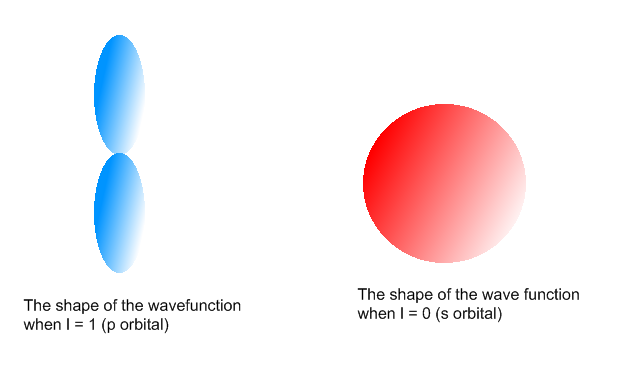To visualize and organize the first three quantum numbers, we can think of them as constituents of a house. In the following image, the roof represents the principal quantum number n, each level represents a subshell l, and each room represents the different orbitals ml in each subshell. The s orbital, because the value of ml can only be 0, can only exist in one plane. The p orbital, however, has three possible values of ml and so it has three possible orientations of the orbitals, shown by Px, Py, and Pz. The pattern continues, with the d orbital containing 5 possible orbital orientations, and f has 7: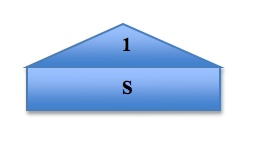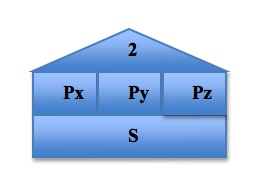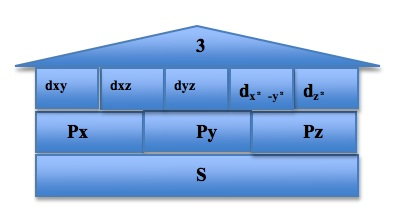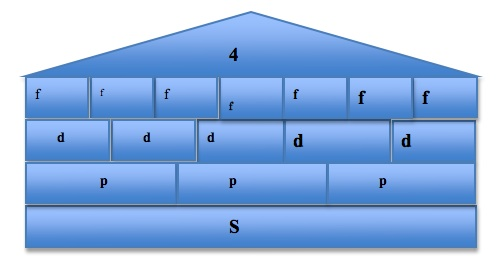Another helpful visual in looking at the possible orbitals and subshells with a set of quantum numbers would be the electron orbital diagram. (For more electron orbital diagrams, see Electron Configurations.) The characteristics of each quantum number are depicted in different areas of this diagram.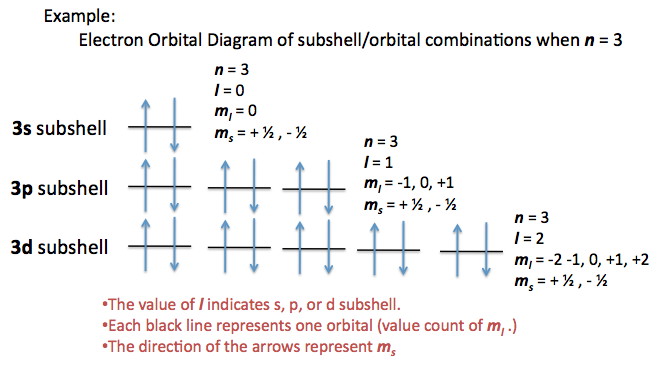## Restrictions

• Pauli Exclusion Principle: In 1926, Wolfgang Pauli discovered that a set of quantum numbers is specific to a certain electron. That is, no two electrons can have the same values for n, l, ml, and ms. Although the first three quantum numbers identify a specific orbital and may have the same values, the fourth is significant and must have opposite spins.
• Hund’s Rule: Orbitals may have identical energy levels when they are of the same principal shell. These orbitals are called degenerate, or “equal energy.” According to Hund’s Rule, electrons fill orbitals one at a time. This means that when drawing electron configurations using the model with the arrows, you must fill each shell with one electron each before starting to pair them up. Remember that the charge of an electron is negative and electrons repel each other. Electrons will try to create distance between it and other electrons by staying unpaired. This further explains why the spins of electrons in an orbital are opposite (i.e. +1/2 and -1/2).
• Heisenberg Uncertainty Principle: According to the Heisenberg Uncertainty Principle, we cannot precisely measure the momentum and position of an electron at the same time. As the momentum of the electron is more and more certain, the position of the electron becomes less certain, and vice versa. This helps explain integral quantum numbers and why n=2.5 cannot exist as a principal quantum number. There must be an integral number of wavelengths (n) in order for an electron to maintain a standing wave. If there were to be partial waves, the whole and partial waves would cancel each other out and the particle would not move. If the particle was at rest, then its position and momentum would be certain. Because this is not so, n must have an integral value. It is not that the principal quantum number can only be measured in integral numbers, it is because the crest of one wave will overlap with the trough of another, and the wave will cancel out.

### References

1. Chang, Raymond. Physical Chemistry for the Biosciences. 2005, University Science Books. pp 427-428.
2. Gillespie, Ronald. Demystifying Introductory Chemistry. The Forum: A Contribution from the Task Force on General Chemistry. 1996: 73;617-622.
3. Petrucci, Ralph. General Chemistry: Principles and Modern Applications, Tenth Edition.

### Problems

1. Suppose that all you know about a certain electron is that its principal quantum number is 3. What are the possible values for the other four quantum numbers?
2. Is it possible to have an electron with these quantum numbers: n=2, l=1, ml=3, ms=1/2? Why or why not?
3. Is it possible to have two electrons with the same n, l, and ml?
4. How many subshells are in principal quantum level n=3?
5. What type of orbital is designated by quantum numbers n=4, l=3, and ml =0?

#### Solutions

• When n=3,  l=0, ml = 0, and ms=+1/2 or -1/2
• l=1, ml = -1, 0, or +1, and ms=+1/2 or -1/2
• l=2, ml = -2, -1, 0, 1 or +2, and ms=+1/2 or -1/2
1. No, it is not possible. ml=3 is not in the range of -l to +l. The value should be be either -1, 0, or +1.
2. Yes, it is possible to have two electrons with the same n, l, and ml. The spin of one electron must be +1/2 while the spin of the other electron must be -1/2.
3. There are three subshells in principal quantum level n=3.
4. Since l=3 refers to the f subshell, the type of orbital represented is 4f (combination of the principal quantum number n and the name of the subshell).

## Contributors

• Anastasiya Kamenko, Tamara Enriquez (UCD), Mandy Lam (UCD)
• Dr. Craig Fisher (Japan Fine Ceramics Center)